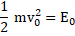# A stationary body explodes into four identical fragments such that three of them fly off mutually perpendicular to each other, each with same KE,The energy of explosion will be a)b)c)d)## Question ID - 100229 :- A stationary body explodes into four identical fragments such that three of them fly off mutually perpendicular to each other, each with same KE,The energy of explosion will be a)b)c)d)3537

 (a) Let the three mutually perpendicular directions be alongand-axis, respectively.WhereandBy linear momentum conservation,ororTotal energyA ball falls under gravity from a height of 10 m with an initial downward velocityIt collides with theground, loses 50%of its energy in collision and then rises back to the same height. Find (i) the initial velocityand (ii) the height to which the ball would rise after collision if the initial velocitywas directed upwards instead of downwards? a)b)c)d)